# afex_plot: Publication Ready Plots for Experimental Designs

#### 2021-01-12

`afex_plot()` visualizes results from factorial experiments combining estimated marginal means and uncertainties associated with the estimated means in the foreground with a depiction of the raw data in the background. Currently, `afex_plots()` supports the following models:

• ANOVAs estimated with `aov_car()`, `aov_ez()`, or `aov_4()` (i.e., objects of class `"afex_aov"`)
• Linear mixed models estimated with `mixed()` (i.e., objects of class `"mixed"`)
• Linear mixed models estimated with `lme4::lmer` (i.e., objects of class `"merMod"`)
• Models with `emmeans` support. For some examples see `vignette("afex_plot_supported_models", package = "afex")`

This document provides an overview of the plots possible with `afex_plot()`. It does so mostly using the `afex_plot()` examples, see `?afex_plot`. We begin by loading `afex` and `ggplot2` which is the package `afex_plot()` uses for plotting. Loading `ggplot2` explicitly is not strictly necessary, but makes the following code nicer. Otherwise, we would need to prepend each call to a function from `ggplot2` needed for customization with `ggplot2::` (as is done in the examples in `?afex_plot`).

We also load the `cowplot` package (introduction) which makes combining plots (with functions `plot_grid()` and `legend()`) very easy. However, loading `cowplot` sets a different theme for `ggplot2` plots than the default grey one. Although I am not a big fan of the default theme with its grey background, we reset the theme globally using `theme_set(theme_grey())` to start with the default behavior if `cowplot` it not attached. Note that `cowplot` also has the cool `draw_plot()` function which allows embedding plots within other plots.

We furthermore will need the following packages, however, we will not attach them directly, but only call a few selected functions using the `package::function` notation.

``````library("afex")
library("ggplot2")
library("cowplot")
theme_set(theme_grey())``````

# Two-Way Within-Subjects ANOVA

We begin with a two-way within-subjects ANOVA using synthetic data from Maxwell and Delaney (2004, p. 547). The data are hypothetical reaction times from a 2 x 3 Perceptual Experiment with factors `angle` with 3 levels and factor `noise` with 2 levels (see `?md_12.1` for a longer description). We first load the data and then fit the corresponding ANOVA.

``````data(md_12.1)
(aw <- aov_ez("id", "rt", md_12.1, within = c("angle", "noise")))``````
``````## Anova Table (Type 3 tests)
##
## Response: rt
##        Effect          df     MSE         F  ges p.value
## 1       angle 1.92, 17.31 3702.02 40.72 *** .390   <.001
## 2       noise        1, 9 8460.00 33.77 *** .387   <.001
## 3 angle:noise 1.81, 16.27 1283.22 45.31 *** .188   <.001
## ---
## Signif. codes:  0 '***' 0.001 '**' 0.01 '*' 0.05 '+' 0.1 ' ' 1
##
## Sphericity correction method: GG``````

The ANOVA shows that both, the two main effect as well as the interaction, are significant. We therefore inspect the pattern underlying the interaction. There exist two different ways of plotting a 2-way interaction. Either of the two variables can be depicted on the x-axis. And before having looked at both cases, it is often not clear which visualization of the interaction is more instructive. Consequently, we plot both next to each other. For this we simply need to exchange which variable is the `x` factor and which is the `trace` factor. We then use `plot_grid()` to plot them next to each other.

## Basic Plot

``p_an <- afex_plot(aw, x = "angle", trace = "noise") ``
``````## Warning: Panel(s) show within-subjects factors, but not within-subjects error bars.
## For within-subjects error bars use: error = "within"``````
``p_na <- afex_plot(aw, x = "noise", trace = "angle")``
``````## Warning: Panel(s) show within-subjects factors, but not within-subjects error bars.
## For within-subjects error bars use: error = "within"``````
``plot_grid(p_an, p_na)  ## try adding: labels = "AUTO"``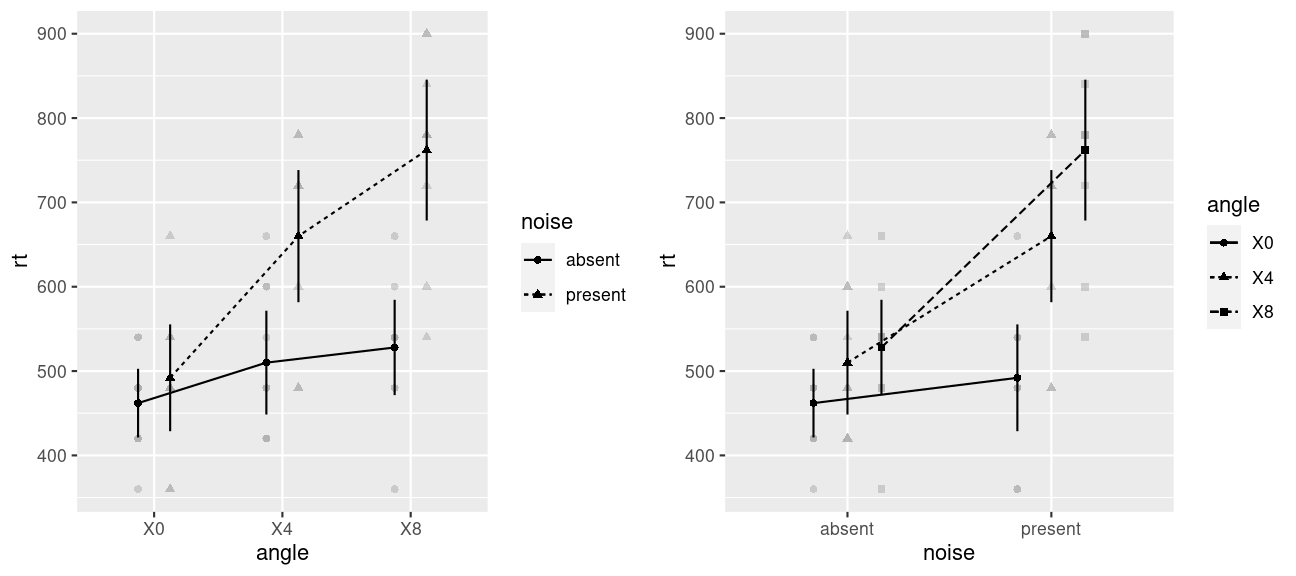Before we can even take a look at the plot, we notice that creating the plots has produced two warnings. These warnings complain that the plots depict within-subject factors, but do not use within-subject error bars. However, the warnings also tell us the solution (i.e., adding `error = "within"`), which we will do in the following. The help page `?afex_plot` contains more information on which type of error bars are appropriate in which situation and how to interpret different type of error bars. For ANOVAs, `afex_plot()` will emit warnings if it thinks the error bars are not appropriate for the chosen factors.

Comparing both plots, my impression is that the plot with `angle` on the `x`-axis tells the clearer story. We can see that when `noise` is `absent` there is hardly any effect of the increase of `angle`. However, if `noise` is `present` an increasing `angle` clearly leads to increased RTs. We therefore use this plot in the following.

## Exploring Graphical Options and Themes

We now produce a new variant of the left plot using more appropriate error bars and change several other graphical details which make the plot publication ready. We use the `factor_levels` argument to `afex_plot()` for renaming the factor levels (for technical reasons the ANOVA functions in `afex` transform all factor levels to proper `R` variable names using `make.names()` which changed the labels from e.g., `4` to `X4`) and the `legend_title` argument for changing the title of the legend. We also change the labels on the `x` and `y` axis.

``````p_an <- afex_plot(aw, x = "angle", trace = "noise", error = "within",
factor_levels = list(angle = c("0°", "4°", "8°"),
noise = c("Absent", "Present")),
legend_title = "Noise") +
labs(y = "RTs (in ms)", x = "Angle (in degrees)")``````
``````## Renaming/reordering factor levels of 'angle':
##   X0 -> 0°
##   X4 -> 4°
##   X8 -> 8°``````
``````## Renaming/reordering factor levels of 'noise':
##   absent -> Absent
##   present -> Present``````

As the additional output shows, changing the factor levels via `factor_levels` emits a `message` detailing old and new factor levels in the form `old -> new`. This message can be suppressed by wrapping the `afex_plot()` call into a `suppressMessages()` call or via `RMarkdown` settings. Note that we could have also used the `factor_levels` argument for changing the order of the factor levels by passing a named character vector (e.g., `factor_levels = list(angle = c(X8 = "8°", X4 = "4°", X0 = "0°"))`). This would change the order either on the x-axis or in the legend.

As said above, I am not a big fan of the default grey theme of `ggplot2` plots. Consequently, we compare a number of different themes for this plot in the following. For all but `ggpubr::theme_pubr()`, we also move the legend to the bottom as this better allows the plot to cover only a single column in a two-column layout. `ggpubr::theme_pubr()` automatically plots the legend on top.

``````plot_grid(
p_an + theme_bw() + theme(legend.position="bottom"),
p_an + theme_light() + theme(legend.position="bottom"),
p_an + theme_minimal() + theme(legend.position="bottom"),
p_an + jtools::theme_nice() + theme(legend.position="bottom"),
p_an + ggpubr::theme_pubr(),
p_an + theme_cowplot() + theme(legend.position="bottom"),
labels = "AUTO"
)  ``````
``````## Registered S3 methods overwritten by 'broom':
##   method            from
##   tidy.glht         jtools
##   tidy.summary.glht jtools``````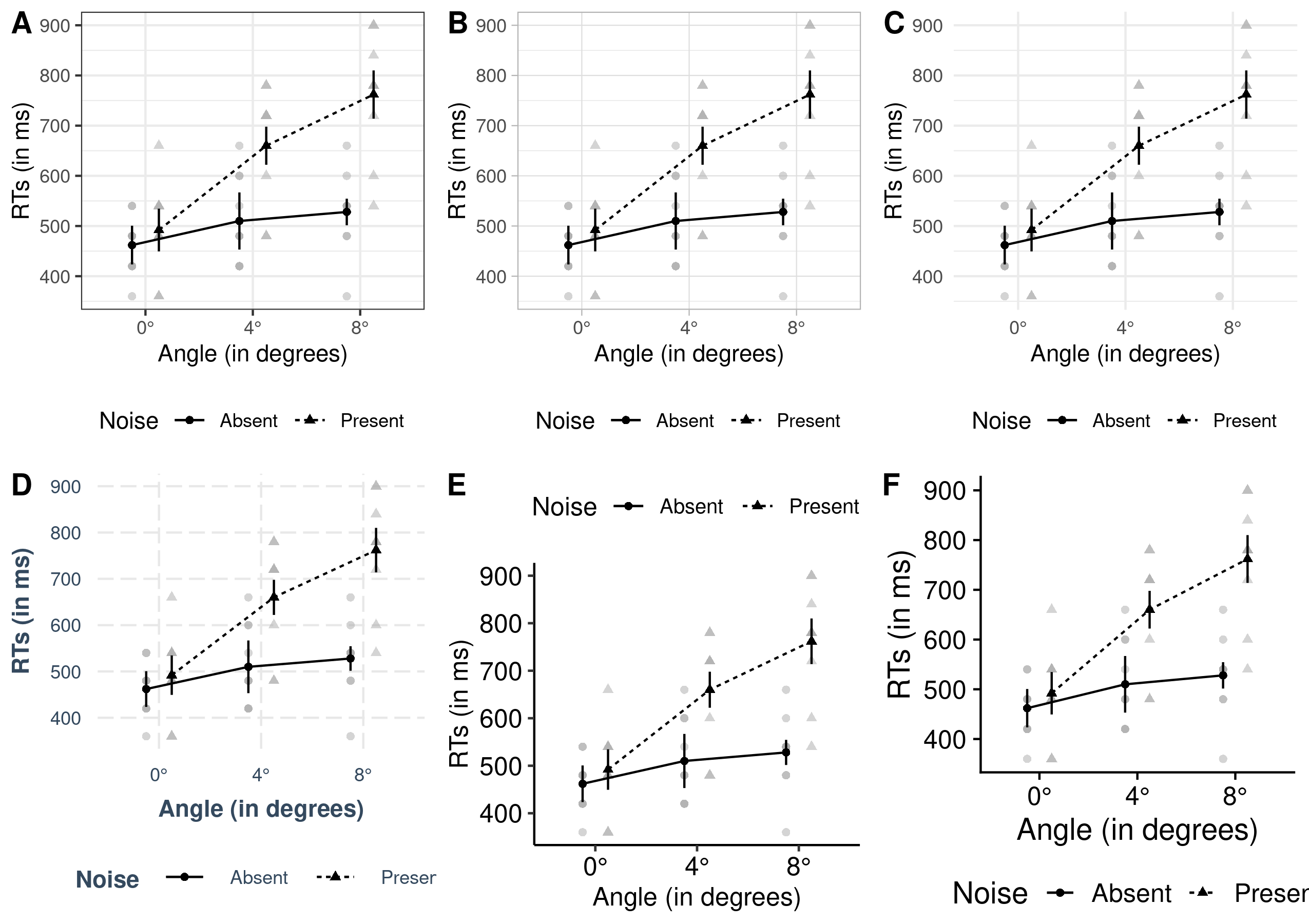The first row, panels A to C, shows themes coming with `ggplot2` and the second row, panels D to F, shows themes from additional packages. In my opinion all of these plots are an improvement above the default grey theme. For the themes coming with `ggplot2`, I really like that those shown here have a reference grid in the background. This often makes it easier to judge the actual values the shown data points have. I know that many people find this distracting, so many of the contributed themes do not have this grid. One thing I really like about the last two themes is that they per default use larger font sizes for the axes labels. One way to achieve something similar for most themes is to change `base_size`.

One general criticism I have with the current plots is that they show too many values on the y-axis. In the following I plot one more variant of this plot in which we change this to three values on the y-axis. We also increase the axes labels and remove the vertical grid lines.

``````p_an +
scale_y_continuous(breaks=seq(400, 900, length.out = 3)) +
theme_bw(base_size = 15) +
theme(legend.position="bottom",
panel.grid.major.x = element_blank())``````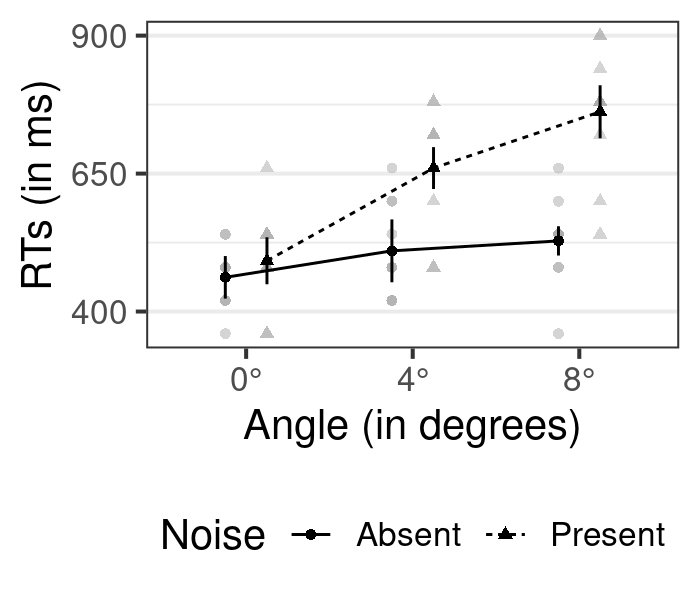We can also set this theme for the reminder of the `R` session with `theme_set()`.

``````theme_set(theme_bw(base_size = 15) +
theme(legend.position="bottom",
panel.grid.major.x = element_blank()))``````

## Saving Plots and Plot Sizes

To get our plot into a publication, we need to export it as a graphics file. I would generally advise against exporting plots via the `RStudio` interface as this is not reproducible. Instead I would use some of the following functions which save the document in the current working directory. Note that following Elsevier guidelines, a single column figure should have a width of 9 cm (~ 3 inch) and a two column figure should have a width of 19 cm (~ 7.5 inch).

For Word or similar documents I would export the plot as a `png` (never `jpg`):

``````ggsave("my_plot.png", device = "png",
width = 9, height = 8, units = "cm",
dpi = 600) ## the larger the dpi, the better the resolution``````

For `LaTeX` I would export as `pdf`:

``````ggsave("my_plot.pdf", device = "pdf",
width = 9, height = 8, units = "cm") ``````

## Data in the Background

`afex_plot()` per default plots the raw data in the background. It does so using an alpha blending of `0.5`. Thus, overlapping points appear darker. Examples of this can be seen in the previous graphs where some data points in the background appear clearly darker than others. The darker points indicate values for which several data points lie exactly on top of each other.

`afex_plot()` provides the possibility to change or alter the graphical primitive, called `geom` in `ggplot2` parlance, used for plotting the points in the background. This offers a vast array of options for handling overlapping points or, more generally, how to display the raw data in the background. I show some of these examples in the following.

The first two variants display only points, whereas the remaining ones use different visualizations of the raw data. Note that depending on the specific variant we change a few further plot options to obtain a visually pleasing result. For example, the `dodge` argument controls the spread of points belonging to different levels of the `trace` factor at each x-axis position.

1. Add jitter on the y-axis to points which avoids perfect overlap.
2. Size of points show number of data points at a given y-axis position: `geom_count`. For this geom, adding a call to `scale_size_area()` can sometimes be beneficial.
3. Violin plot: `geom_violin`
4. Box plot: `geom_boxplot`. Note that for this plot we have added `linetype = 1` to `data_arg`, which avoids that the outline of the box plots is affected by the linetype mapping (this is in contrast with the violin plot).
5. Display points using a bee swarm plot, which displaces overlapping points on the x-axis: `ggbeeswarm::geom_beeswarm`
6. Display points using a variant of the bee swarm plot that even works in case of many data points: `ggbeeswarm::geom_quasirandom`
7. Combine box plot with jittered points: `ggpol::geom_boxjitter`
``````p1 <- afex_plot(aw, x = "noise", trace = "angle", error = "within", dodge = 0.3,
data_arg = list(
position =
ggplot2::position_jitterdodge(
jitter.width = 0,
jitter.height = 10,
dodge.width = 0.3  ## needs to be same as dodge
),
color = "darkgrey"))
p2 <- afex_plot(aw, x = "noise", trace = "angle", error = "within", dodge = 0.5,
data_geom = geom_count)
p3 <- afex_plot(aw, x = "noise", trace = "angle", error = "within",
data_geom = geom_violin,
data_arg = list(width = 0.5))
p4 <- afex_plot(aw, x = "noise", trace = "angle", error = "within",
data_geom = geom_boxplot,
data_arg = list(width = 0.3, linetype = 1))
p5 <- afex_plot(aw, x = "noise", trace = "angle", error = "within", dodge = 0.5,
data_geom = ggbeeswarm::geom_beeswarm,
data_arg = list(
dodge.width = 0.5,  ## needs to be same as dodge
cex = 0.8,
color = "darkgrey"))
p6 <- afex_plot(aw, x = "noise", trace = "angle", error = "within", dodge = 0.5,
data_geom = ggbeeswarm::geom_quasirandom,
data_arg = list(
dodge.width = 0.5,  ## needs to be same as dodge
color = "darkgrey"))
p7 <- afex_plot(aw, x = "noise", trace = "angle", error = "within", dodge = 0.7,
data_geom = ggpol::geom_boxjitter,
data_arg = list(
width = 0.5,
jitter.params = list(width = 0, height = 10),
outlier.intersect = TRUE),
point_arg = list(size = 2.5),
error_arg = list(size = 1.5, width = 0))
plot_grid(p1, p2, p3, p4, p5, p6, p7, ncol = 2, labels = 1:7)  ``````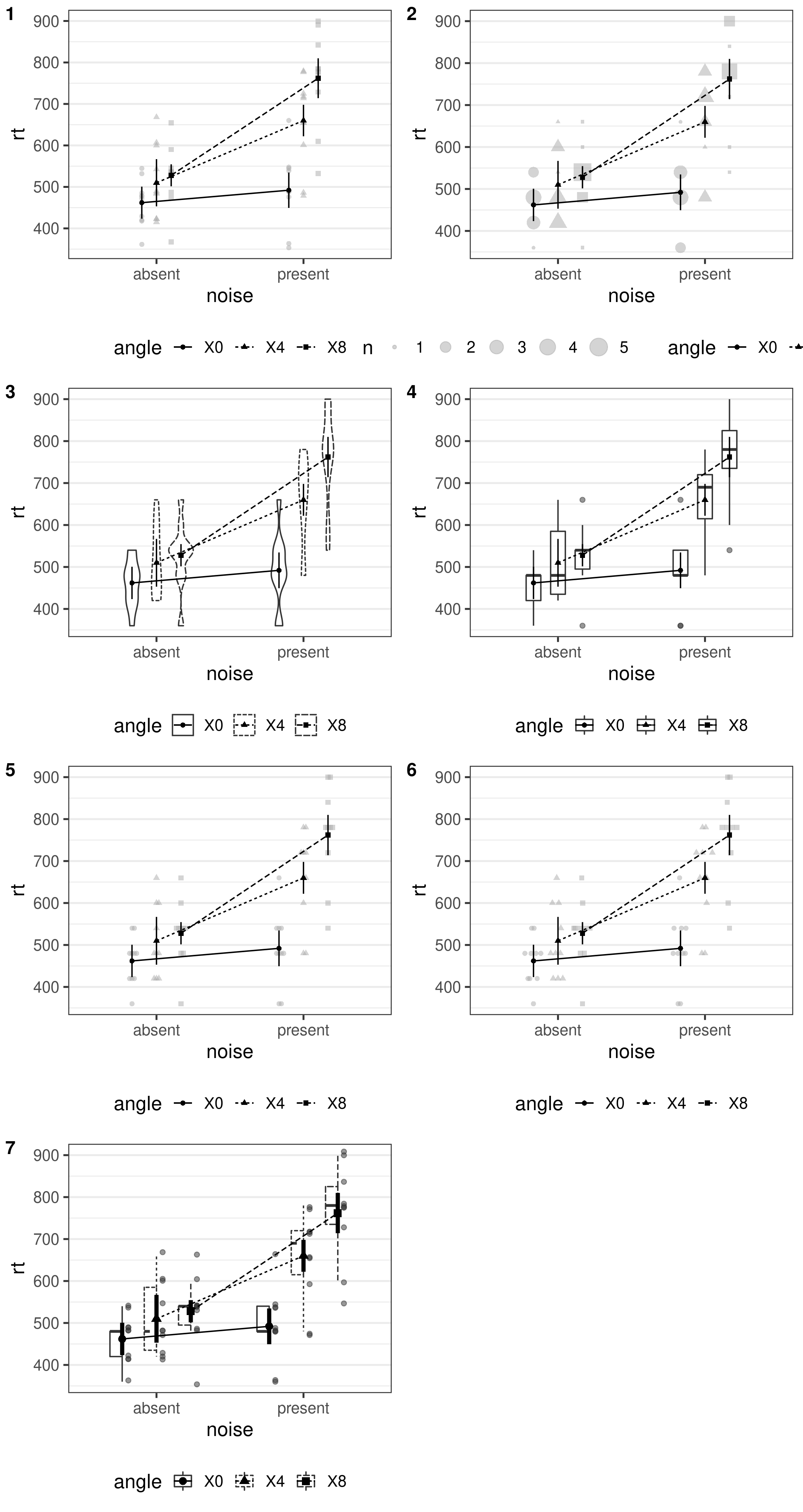So far, all plots were shown in black and white only. However, it is easy to include color. We do so for plots 2 to 5 from above. To achieve this, we have to change the value of the `mapping` argument.

``````p2 <- afex_plot(aw, x = "noise", trace = "angle", error = "within", dodge = 0.5,
mapping = c("shape", "color"),
data_geom = ggbeeswarm::geom_beeswarm,
data_arg = list(
dodge.width = 0.5,  ## needs to be same as dodge
cex = 0.8))
p3 <- afex_plot(aw, x = "noise", trace = "angle", error = "within",
mapping = c("linetype", "shape", "fill"),
data_geom = ggplot2::geom_violin,
data_arg = list(width = 0.5))
p4 <- afex_plot(aw, x = "noise", trace = "angle", error = "within",
mapping = c("shape", "fill"),
data_geom = ggplot2::geom_boxplot,
data_arg = list(width = 0.3))
p5 <- afex_plot(aw, x = "noise", trace = "angle", error = "within", dodge = 0.7,
mapping = c("shape", "fill"),
data_geom = ggpol::geom_boxjitter,
data_arg = list(
width = 0.5,
jitter.params = list(width = 0, height = 10),
outlier.intersect = TRUE),
point_arg = list(size = 2.5),
line_arg = list(linetype = 0),
error_arg = list(size = 1.5, width = 0))
plot_grid(p2, p3, p4, p5, ncol = 2, labels = 2:5) ``````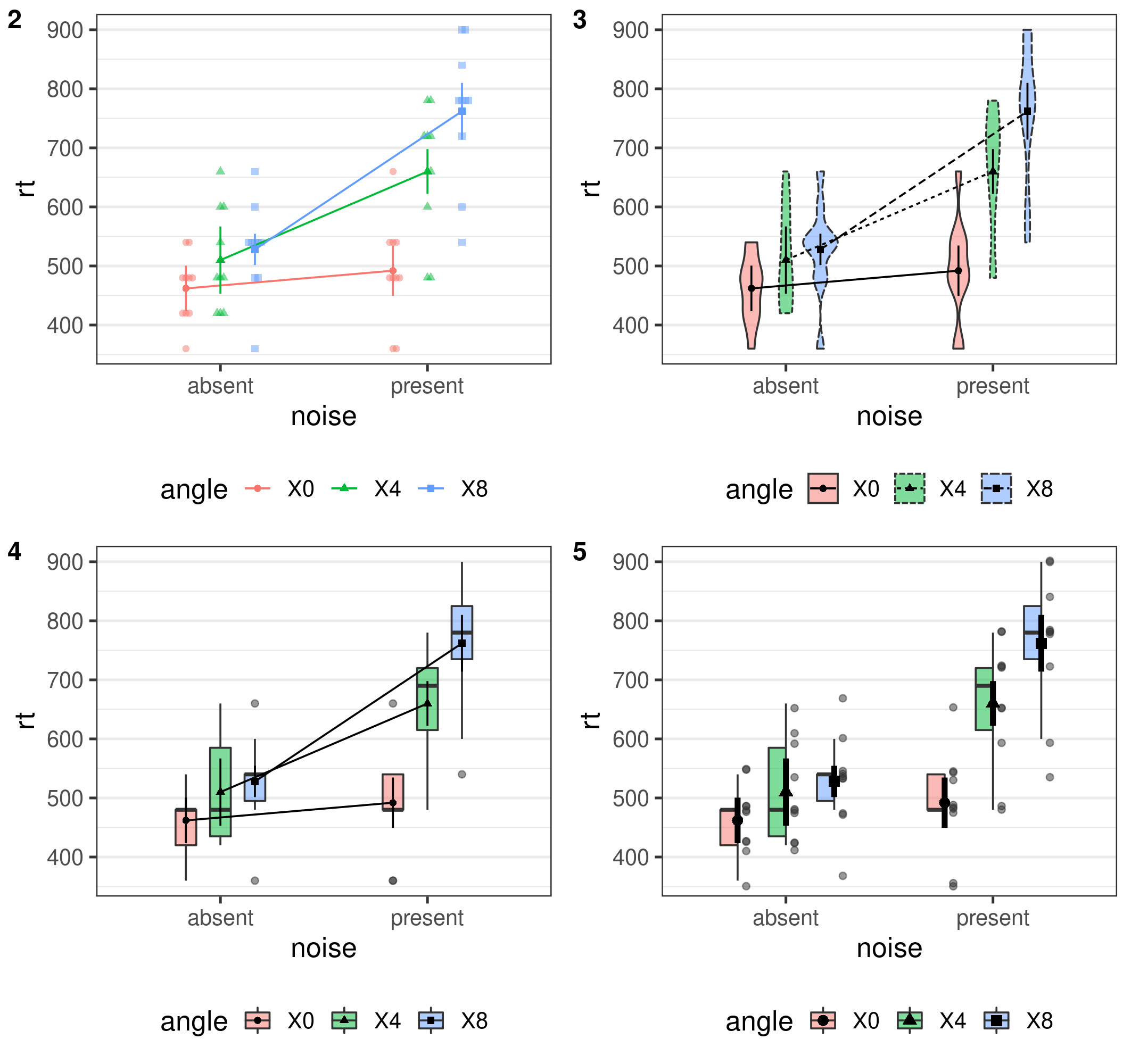## Plotting Order and Error Bars

For graphical element in the foreground, `afex_plot` first plots all graphical elements belonging to the same factor level before plotting graphical elements belonging to different factor levels. This provides a consistent graphical impression for each factor level that is particularly relevant in case color is mapped.

In case we have overlapping lines and error bars or use thick lines, we sometimes do not want that the error bars also receives different line types. In this case, we can simply pass `linetype = 1` to `error_arg` to overwrite the corresponding mapping. This is shown in the right plot.

``````p1 <- afex_plot(aw, x = "noise", trace = "angle", mapping = "color",
error = "within",
point_arg = list(size = 5), line_arg = list(size = 2),
error_arg = list(size = 2))
p2 <- afex_plot(aw, x = "noise", trace = "angle",
mapping = c("color", "shape", "linetype"),
error = "within",
point_arg = list(size = 5), line_arg = list(size = 2),
error_arg = list(size = 2, width = 0, linetype = 1))
plot_grid(p1, p2, ncol = 2)``````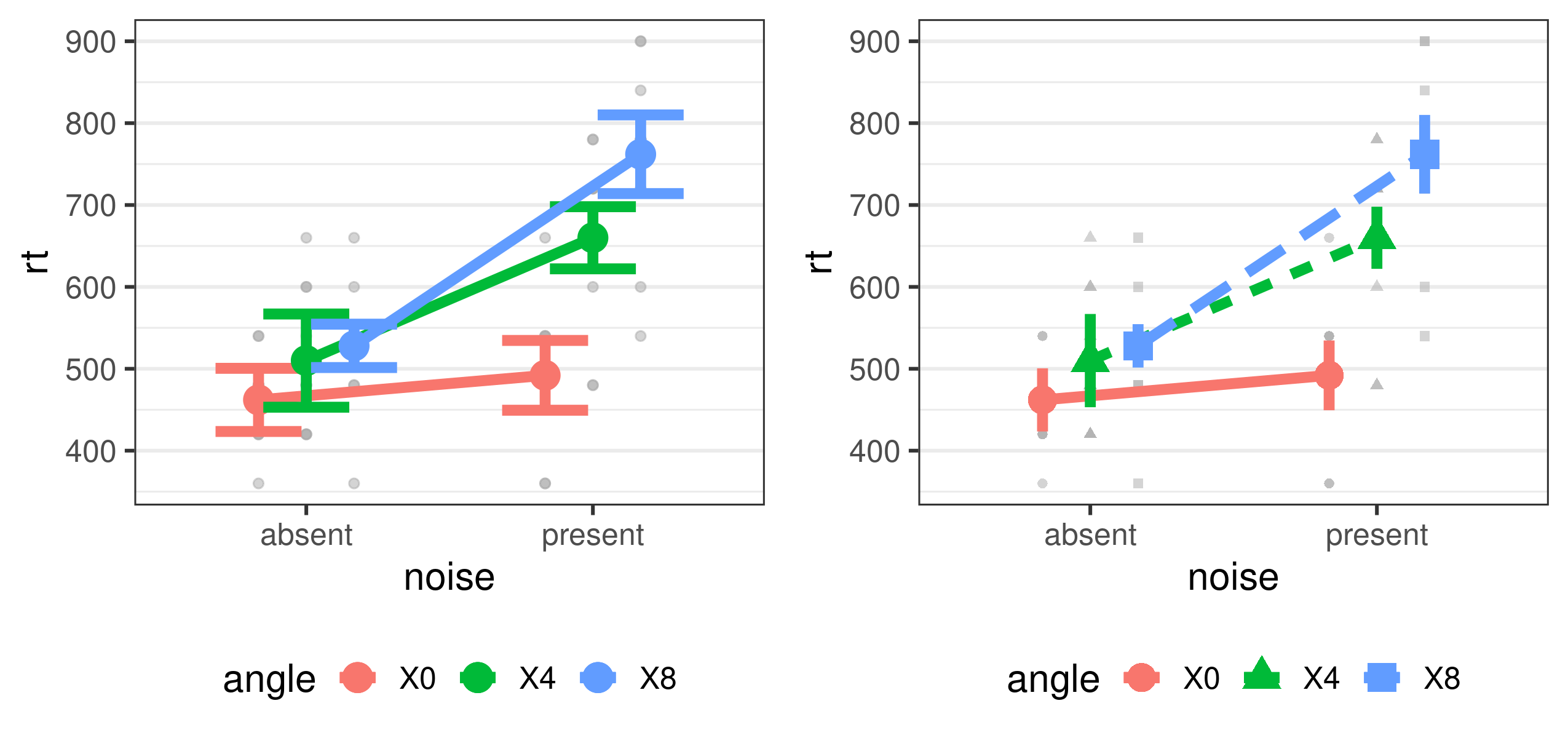## One-way Plots Without Trace Factor

If `afex_plot` is called without a trace factor, a one-way plot is created. We can customize this plot in very much the same way. Per default a one-way plot contains a legend if `mapping` is not empty (i.e., `""`). We show this legend for the left plot, but suppress it for the right one.

``````po1 <- afex_plot(aw, x = "angle", mapping = "color", error = "within",
data_arg = list(),
point_arg = list(size = 2.5),
error_arg = list(size = 1.5, width = 0.05))
po2 <- afex_plot(aw, x = "angle", error = "within",
data_geom = ggpol::geom_boxjitter,
mapping = "fill", data_alpha = 0.7,
data_arg = list(
width = 0.6,
jitter.params = list(width = 0.05, height = 10),
outlier.intersect = TRUE
),
point_arg = list(size = 2.5),
error_arg = list(size = 1.5, width = 0.05)) +
theme(legend.position="none")
plot_grid(po1, po2) ``````